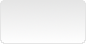# Javascript - Importing HTML blocks, fetch## Introduction

For relatively static HTML blocks (headers, footers…), we search an easy way to import HTML code from files.

There was an easy way, like importing CSS, JS files :

``<link rel="import" href="footer.html">``

BUT, this specification has been deprecated. It does not work anymore since Chrome 73. It may still work in some browsers, its use is discouraged since it could be removed at any time.

What is the alternative ? Use the javascript `fetch` method.

In the example, we want to include the footer HTML data from a file called `footer.inc` :

`footer.inc`
``````<div>
<div>
<nav class="inline-right pipe">
<ul>
</ul>
</nav>
</div>
</div>``````

## The fetch method

Create a function which will load the data after the `DOMContentLoaded` event :

``````loadfooter = () => {
fetch('./include/footer.inc')
.then ( (r) => { return r.text();  } )
.then ( (s) => {
p= new DOMParser();
d = p.parseFromString(s,'text/html') ;
});
};

• The `text()` method returns the content of the file in a string variable.
• A new DOM object is built from the returned string using `parseFromString()`.

The DOM object structure is then the following :

``````#document
<html>
<body>
<div>
…
</div>
</body>
</html>``````

The `div` block to be inserted is then extracted from the DOM object and added in the existing `footer` block in the document :

``````loadfooter = () => {

fetch('./include/fr/footer.inc')
.then ( (r) => {  return r.text();  } )
.then ( (s) => {
p= new DOMParser();
d = p.parseFromString(s,'text/html') ;

if ((f = d.body.querySelector('div')) !== null) {
document.querySelector('footer').append(f);
}
});
};``````
The element is moved from the DOM object to the document. Use `clone` method if we don’t want the element to be removed from the DOM object for further processing.

## Templates

The `fetch` and `parseFromString` methods are very useful to import template files. The element can be indeed enriched and formatted before inserting it into the final document.

``````loadfooter = () => {

fetch('./include/fr/footer.inc')
.then ( (r) => {  return r.text();  } )
.then ( (s) => {
p= new DOMParser();
d = p.parseFromString(s,'text/html') ;

if ((f = d.body.querySelector('div')) !== null) {

f.querySelector(`span[id="footer-org"]`)
.append(document.createTextNode('SQLPAC'));

document.querySelector('footer').append(f);
}
});
};``````